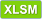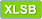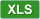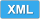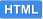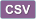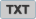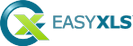# How to read XLS file in C++.NET

 View Excel fileCopy code ``````/*---------------------------------------------------------------------------- | Tutorial 37 | | This tutorial shows how to read an Excel XLS file in C++.NET (the | XLS file generated by Tutorial 28 as base template), modify | some data and save it to another XLS file (Tutorial37 - read XLS file.xls). ---------------------------------------------------------------------------*/ #include "stdafx.h" using namespace System; using namespace EasyXLS; using namespace System::Data; using namespace System::IO; int main() { Console::WriteLine("Tutorial 37\n----------\n"); // Create an instance of the class that reads Excel files ExcelDocument ^workbook = gcnew ExcelDocument(); // Read XLS file Console::WriteLine("Reading file C:\\Samples\\Tutorial28.xls.\n"); if (workbook->easy_LoadXLSFile("C:\\Samples\\Tutorial28.xls")) { // Get the table of data for the second worksheet ExcelWorksheet ^xlsSecondTab = safe_cast(workbook->easy_getSheetAt(1)); ExcelTable ^xlsSecondTable = xlsSecondTab->easy_getExcelTable(); // Write some data to the second sheet xlsSecondTable->easy_getCell("A1")->setValue("Data added by Tutorial37"); for (int column=0; column<5; column++) { xlsSecondTable->easy_getCell(1, column)->setValue(String::Concat("Data ", (column + 1).ToString())); } // Export the new XLS file Console::WriteLine("Writing file C:\\Samples\\Tutorial37 - read XLS file.xls."); workbook->easy_WriteXLSFile("C:\\Samples\\Tutorial37 - read XLS file.xls"); // Confirm export of Excel file String ^sError = workbook->easy_getError(); if (sError->Equals("")) Console::Write("\nFile successfully created. Press Enter to Exit..."); else Console::Write(String::Concat("\nError encountered: ", sError, "\nPress Enter to Exit...")); } else { Console::WriteLine(String::Concat("\nError reading file C:\\Samples\\Tutorial28.xls \n", workbook->easy_getError(), "\nPress Enter to Exit...")); } // Dispose memory delete workbook; Console::ReadLine(); return 0; } ``````

C++ without .NET Framework
This tutorial is valid for C++ applications that can use .NET Framework. For applications that does not have .NET Framework integrated, a similar code sample is available.

#### EasyXLS Excel libraries: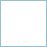##### Excel Library for C++.NET
full .NET version to import, export or convert Excel files